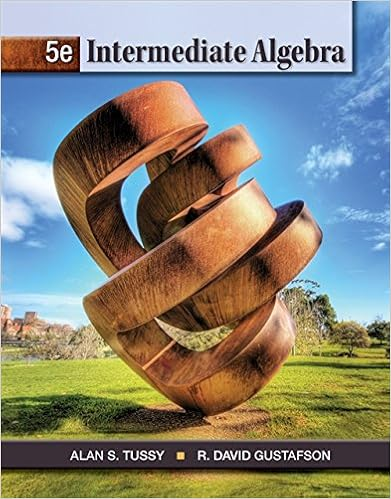# assignment sheet-WA10_MAT-115-mar18.docx - MAT-115...

• Homework Help
• 8
• 100% (15) 15 out of 15 people found this document helpful

This preview shows page 1 - 2 out of 8 pages.

##### We have textbook solutions for you!
The document you are viewing contains questions related to this textbook.The document you are viewing contains questions related to this textbook.
Chapter 9 / Exercise 12
Intermediate Algebra
Tussy/GustafsonExpert Verified
MAT-115: INTERMEDIATE ALGEBRAWritten Assignment 1010.1: Finding Composite and Inverse FunctionsSolve.1.If f(x)=3x+2and g(x)=2x+5, find: (gf)(5)
2.If f(x)=2x4and g(x)=2x+8, find:
3)
3)
4.Determine if the set of ordered pairs is a function. If it is a function, is it one-to-one?
5.Determine if the set of ordered pairs is a function. If it is a function, is it one-to-one?
6.Determine if the set of ordered pairs is a function. If it is a function, is it one-to-one?
##### We have textbook solutions for you!
The document you are viewing contains questions related to this textbook.The document you are viewing contains questions related to this textbook.
Chapter 9 / Exercise 12
Intermediate Algebra
Tussy/GustafsonExpert Verified
•••